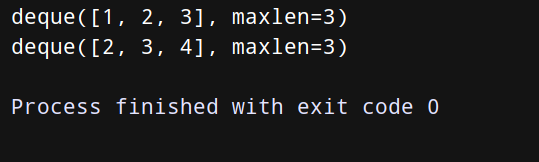﻿ Python中的元组(Tuple)操作实例详解_python_脚本之家
python# Python中的元组(Tuple)操作实例详解

Python 元组和列表很像,也是一个包含多个元素的集合,下面这篇文章主要给大家介绍了关于Python中元组(Tuple)操作的相关资料,文中通过实例代码介绍的非常详细,需要的朋友可以参考下

## 1.元组的 创建 && 访问

### （1）元组的创建：

```tuple1 = ('xiaoming', 'xiaohong', 18, 21)
tuple2 = (1, 2, 3, 4, 5)

# 而且——是可以创建空元组哦！
tuple3 = ()

# 小注意——如果你创建的元组只包含一个元素时，也不要忘记在元素后面加上逗号。让其识别为一个元组：
tuple4 = (22, )
```

### （2）访问：

```tuple1 = ('xiaoming', 'xiaohong', 18, 21)
tuple2 = (1, 2, 3, 4, 5)

# 显示元组中索引为1的元素的值
print("tuple1:", tuple1)

# 显示元组中索引从1到3的元素的值
print("tuple2[1:3]:", tuple2[1:3])
```## 2.元组的 修改 && 删除

### （1）元组的修改：

```tuple1 = ('xiaoming', 'xiaohong', 18, 21)
tuple2 = (1, 2, 3, 4, 5)

tuple_new = tuple1 + tuple2
print(tuple_new)
```### （2）元组的删除：

```tuple1 = ('xiaoming', 'xiaohong', 18, 21)

print(tuple1)		# 正常打印tuple1

del tuple1

print(tuple1)		# 因为上面删除了tuple1，所以再打印会报错哦！
```## 3.元组的内置方法

• len(tuple)：计算元组元素个数；
• max(tuple)：返回元组中元素的最大值；
• min(tuple)：返回元组中元素的最小值；
• tuple(seq)：将列表转换为元组。

## 4.将序列分解为单独的变量

（1）

Python允许将一个包含N个元素的元组或序列分别为N个单独的变量。这是因为Python语法允许任何序列/可迭代对象通过简单的赋值操作分解为单独的变量，唯一的要求是变量的总数和结构要与序列相吻合。

```tuple1 = (18, 22)
x, y = tuple1
print(x)
print(y)

tuple2 = ['xiaoming', 33, 19.8, (2012, 1, 11)]
name, age, level, date = tuple2
print(name)
print(date)
``````records = [
('AAA', 1, 2),
('BBB', 'hello'),
('CCC', 5, 3)
]

def do_foo(x, y):
print('AAA', x, y)

def do_bar(s):
print('BBB', s)

for tag, *args in records:
if tag == 'AAA':
do_foo(*args)
elif tag == 'BBB':
do_bar(*args)

line = 'guan:ijing234://wef:678d:guan'
uname, *fields, homedir, sh = line.split(':')
print(uname)
print(*fields)
print(homedir)
print(sh)
```（2）

```from _collections import deque

q = deque(maxlen=3)
q.append(1)
q.append(2)
q.append(3)
print(q)
q.append(4)
print(q)
``````from _collections import deque

def search(lines, pattern, history=5):
previous_lines = deque(maxlen=history)

for line in lines:
if pattern in line:
yield line, previous_lines
previous_lines.append(line)
# Example use on a file
if __name__ == '__main__':
with open('123.txt') as f:
for line, prevlines in search(f, 'python', 5):
for pline in prevlines:	# 包含python的行
print(pline)  # print (pline, end='')
# 打印最后检查过的N行文本
print(line)  # print (pline, end='')
```

123.txt：

pythonpythonpythonpythonpythonpythonpython

python

python## 5.实现优先级队列

```import heapq
class PriorityQueue:
def __init__(self):
self._queue = []
self._index = 0

def push(self, item, priority):
heapq.heappush(self._queue, (-priority, self._index, item))
self._index += 1

def pop(self):
return heapq.heappop(self._queue)[-1]

class Item:
def __init__(self, name):
self.name = name

def __repr__(self):
return 'Item({!r})'.format(self.name)

q = PriorityQueue()
q.push(Item('AAA'), 1)
q.push(Item('BBB'), 4)
q.push(Item('CCC'), 5)
q.push(Item('DDD'), 1)
print(q.pop())
print(q.pop())
print(q.pop())
```

```import heapq
class PriorityQueue:
def __init__(self):
self._queue = []
self._index = 0

def push(self, item, priority):
heapq.heappush(self._queue, (-priority, self._index, item))
self._index += 1

def pop(self):
return heapq.heappop(self._queue)[-1]

class Item:
def __init__(self, name):
self.name = name

def __repr__(self):
return 'Item({!r})'.format(self.name)

# ①
a = Item('AAA')
b = Item('BBB')
#a < b  错误
a = (1, Item('AAA'))
b = (5, Item('BBB'))
print(a < b)
c = (1, Item('CCC'))
#② a < c 错误
# ③
a = (1, 0, Item('AAA'))
b = (5, 1, Item('BBB'))
c = (1, 2, Item('CCC'))
print(a < b)
# ④
print(a < c)
````在上述代码中，因为在①~②中没有添加所以，所以当两个元组的优先级相同时会出错；而在③~④中添加了索引，这样就不会出错了！`